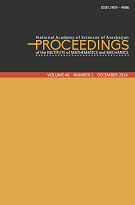P R O C E E D I N G S
of the
Institute of Mathematics and Mechanics

 ISSN 2409-4986 (Print) ISSN 2409-4994 (Online)

## Volume 43, Issue 1

A survey on multinormed von Neumann algebras
by Anar Dosi
367
On nonlinear beam equation with indefinite weight
by Ziyatkhan S. Aliyev and Khayala B. Mehtiyeva
6878
Higher order conditions in nondifferentiable programming problems
7997
Cubature formula for a class of surface integrals generated by weakly-singular integrals
by Rahib J. Heydarov
98104
On the Tietze extension theorem in soft topological spaces
by Cigdem Gunduz Aras and Sadi Bayramov
105115
On $\phi$--solvability of a class of boundary value problems for an operator-differential equation in Hilbert space
by Sabir S. Mirzoev and Sevinj F. Babayeva
116122
Travelling-wave solutions for Klein-Gordon and Helmholtz equations on Cantor sets
by Xiao-Jun Yang, Yusif S. Gasimov, Feng Gao and Natavan Allahverdiyeva
123131
On discreteness of negative part of spectrum and estimates for the number of eigen values of second order equation with operator coefficients on the semi-axis
by Hamidulla I. Aslanov and Nigar A. Gadirli
132145
On the well-posedness of the Cauchy problem for a class of differential equations with distributed delay and the continuous initial condition
Problems of $g$-lifts